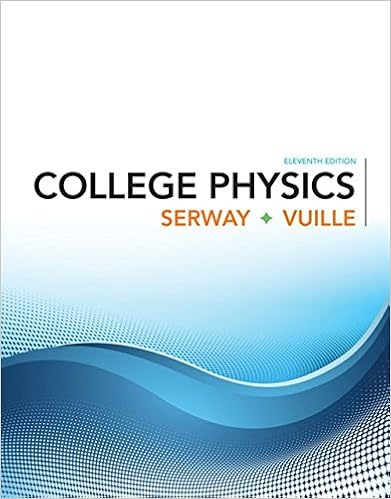# An ordinary workshop grindstone has a radius of 750

• Homework Help
• 9
• 95% (40) 38 out of 40 people found this document helpful

This preview shows page 2 - 5 out of 9 pages.

##### We have textbook solutions for you!
The document you are viewing contains questions related to this textbook.The document you are viewing contains questions related to this textbook.
Chapter 7 / Exercise 25
College Physics
Serway/VuilleExpert Verified
14. An ordinary workshop grindstone has a radius of 7.50 cm and rotates at 6500 rev/min. (a) Calculate the magnitude of the centripetal acceleration at
##### We have textbook solutions for you!
The document you are viewing contains questions related to this textbook.The document you are viewing contains questions related to this textbook.
Chapter 7 / Exercise 25
College Physics
Serway/VuilleExpert Verified
.
(b) What is the linear speed of a point on its edge? s
15. Helicopter blades withstand tremendous stresses. In addition to supporting the weight of a helicopter, they are spun at rapid rates and experience large centripetal accelerations, especially at the tip. (a) Calculate the magnitude of the centripetal acceleration at the tip of a 4.00 m long helicopter blade that rotates at 300 rev/min. (b) Compare the linear speed of the tip with the speed of sound (taken to be 340 m/s).
18. Verify that the linear speed of an ultracentrifuge is about 0.50 km/s, and Earth in its orbit is about 30 km/s by calculating: (a) The linear speed of a point on an ultracentrifuge 0.100 m from its center, rotating at 50,000 rev/min. (b) The linear speed of Earth in its orbit about the Sun (use data from the text on the radius of Earth’s orbit and approximate it as being circular).
•••